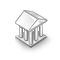Interesting script?
So post a link to it -
let others appraise it
You liked the script? Try it in the MetaTrader 5 terminal# MovingAverages - library for MetaTrader 5

|
Views:
15248
Rating:
Published:
2010.02.04 11:51
Updated:
2016.11.22 07:32

The MovingAverages library is a part of Standard package of MetaTrader 5 client terminal.

The library contains functions for calculation of different types of moving averages. Totally, there are 8 functions that can be divided into 2 groups of functions of the same type, each containing 4 of them.

The first group contains functions that receive an array and simply return a value of a moving average at a specified position:

These functions are intended for obtaining the value of an average once for an array, and are not optimized for multiple calls. If you need to use a function from this group in a loop (to calculate values of an average and further write each calculated value into an array), you'll have to organize an optimal algorithm.

The second group of functions is intended for filling out the recipient array by values of a moving average based on the array of initial values:

• SimpleMAOnBuffer() - fills out the output array buffer[] by values of a simple average from the price[] array;
• ExponentialMAOnBuffer() - fills out the output array buffer[] by values of an exponential average from the price[] array;
• SmoothedMAOnBuffer() - fills out the output array buffer[] by values of a smoothed average from the price[] array;
• LinearWeightedMAOnBuffer() - fills out the output array buffer[] by values of a linear weighted average from the price[] array.

Functions:

```//+------------------------------------------------------------------+
//|                                               MovingAverages.mqh |
//|                        Copyright 2009, MetaQuotes Software Corp. |
//|                                              https://www.mql5.com |
//+------------------------------------------------------------------+
#property copyright "2009, MetaQuotes Software Corp."
//+------------------------------------------------------------------+
//| Simple Moving Average                                            |
//+------------------------------------------------------------------+
double SimpleMA(const int position,const int period,const double &price[])
//+------------------------------------------------------------------+
//| Exponential Moving Average                                       |
//+------------------------------------------------------------------+
double ExponentialMA(const int position,const int period,const double prev_value,const double &price[])
//+------------------------------------------------------------------+
//| Smoothed Moving Average                                          |
//+------------------------------------------------------------------+
double SmoothedMA(const int position,const int period,const double prev_value,const double &price[])
//+------------------------------------------------------------------+
//| Linear Weighted Moving Average                                   |
//+------------------------------------------------------------------+
double LinearWeightedMA(const int position,const int period,const double &price[])

//+------------------------------------------------------------------+
//| Simple moving average on price array                             |
//+------------------------------------------------------------------+
int SimpleMAOnBuffer(const int rates_total,const int prev_calculated,const int begin,
//+------------------------------------------------------------------+
//| Exponential moving average on price array                        |
//+------------------------------------------------------------------+
int ExponentialMAOnBuffer(const int rates_total,const int prev_calculated,const int begin,
const int period,const double& price[],double& buffer[])
//+------------------------------------------------------------------+
//| Smoothed moving average on price array                           |
//+------------------------------------------------------------------+
int SmoothedMAOnBuffer(const int rates_total,const int prev_calculated,const int begin,
const int period,const double& price[],double& buffer[])
//+------------------------------------------------------------------+
//| Linear Weighted moving average on price array                    |
//+------------------------------------------------------------------+
int LinearWeightedMAOnBuffer(const int rates_total,const int prev_calculated,const int begin,
const int period,const double& price[],double& buffer[])
```

Example:

For examples of its use see article "MQL5: Create Your Own Indicator"

Translated from Russian by MetaQuotes Ltd.
Original code: https://www.mql5.com/ru/code/77Triple Exponential Average (TRIX)

It's an oscillator of the overbought/oversold market conditions. It can also be used as the Momentum indicator. Triple smoothing is used for removing the cyclic components in price movements with the period less than that of TRIX.Variable Index Dynamic Average (VIDYA)

This oscillator measures the ratio between the sum of positive increments and sum of negative increments for a certain period.LoongClock

A very simple sample of clockErrorDescription

The library contains functions that returns description of runtime error codes and trade server return codes.